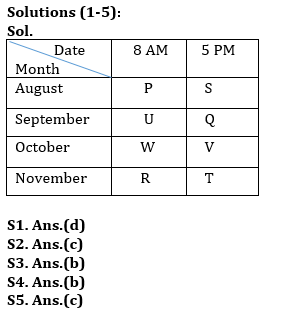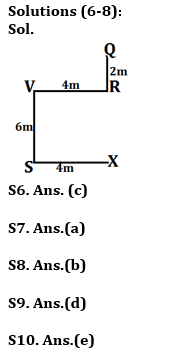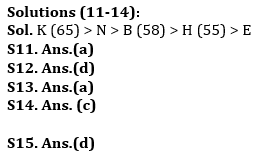Latest Banking jobs   »   Reasoning Ability Quiz For ESIC- UDC,...

# Reasoning Ability Quiz For ESIC- UDC, Steno, MTS Prelims 2022- 13th January

Directions (1-5): Study the following information carefully and answer the given questions:
Eight students are appearing in an examination in four different months- August to November in two different shifts- 8 AM and 5 PM. Only two persons give exam in between W and T, whose exam is in the month which have even number of days. P gives exam in the month having 31 days. S gives exam in evening shift but before W. Three persons give exam between U and R. W gives exam before T. V gives an exam in evening shift of the month which have odd number of days but after W. U gives the exam in the month having 30 days but not in November. Only two persons give exam between P and Q.

Q1. Who among the following appears for exam in October in evening shift?
(a) T
(b) Q
(c) S
(d) V
(e) None of these

Q2. T appears in exam in which of the following month?
(a) October
(b) September
(c) November
(d) August
(e) Can’t determine

Q3. Who among the following appears in examination immediate before Q?
(a) V
(b) U
(c) S
(d) R
(e) None of these

Q4. Which of the following statement is not true about R?
(a) R appears for exam in morning shift
(b) R appears for the exam in the month which have odd numbers of days
(c) T appears in exam immediate after R
(d) Only two persons appear in exam between R and Q
(e) Both (b) and (d)

Q5. Who among the following appears in examination in October at 8 AM?
(a) P
(b) R
(c) W
(d) U
(e) None of these

Directions (6-8): Read the following information carefully and answer the given questions.
Arya starts waking from a point X to point S in the west direction and covers 4 km. He then turned to his right and walks for 6 km to reach at a point V, from here he again took a right turn and walks for 4 km to reach at a point R. He finally turned to his left and walks for another 2 km to reach at a final point Q.

Q6. If point T is in the midway of point V and point R, then in which direction point Q is with respect to point T?
(a) North
(b) South West
(c) North East
(d) North West
(e) None of these

Q7. If point W is just in the middle of point S and point V, then what is the shortest distance between point X and point W?
(a) 5 km
(b) 7 km
(c) 6 km
(d) 4 km
(e) None of these

Q8. What is the direction of point R with respect to point S?
(a) West
(b) North-East
(c) South-West
(d) South
(e) None of these

Q9. If in the number 78364925, 1 is added to each of the even digit and 1 is subtracted in each odd digit, then all digits are arranged in ascending order from left to right. Then which of the following digit is 5th from the right end?
(a) 3
(b) 7
(c) 6
(d) 5
(e) 8

Q10. If all the letters in the word INSPIRATION are arranged in alphabetical order from left to right in such a way that vowels are arranged first followed by consonants, then how many letters are there in between O and S after the arrangement?
(a) two
(b) one
(c) none
(d) three
(e) four

Directions (11-14): Read the following information carefully and answer the given questions.
There are five friends, B, E, H, K and N, each of them got different number of chocolates distributed in a birthday party. K got 7 more chocolates than B. N got more chocolates than three people. E got lowest number of chocolates amongst all. H got less chocolates than N. E got number of chocolates, which is an odd number. K got 65 chocolates. The person who got second lowest number of chocolates got 3 less chocolates than B.

Q11. Who among the following got the third lowest number of chocolates?
(a) B
(b) H
(c) K
(d) N
(e) Can’t determined

Q12. What will be the possible number of chocolates N have?
(a) 66
(b) 57
(c) 58
(d) 62
(e) 71

Q13. Who among the following got more chocolate than N?
(a) K
(b) E
(c) B
(d) E
(e) Can’t determined

Q14. What is the possible number of chocolate E got?
(a) 52
(b) 56
(c) 53
(d) 57
(e) 60

Q15.How many pairs of letters are there in the word ‘ECONOMIST’, each of which have as many letters between then in the word as they have between then in the English alphabet?
(a) Four
(b) One
(c) Two
(d) Three
(e) None

Solutions#### Congratulations!Union Budget 2023-24: Free PDF